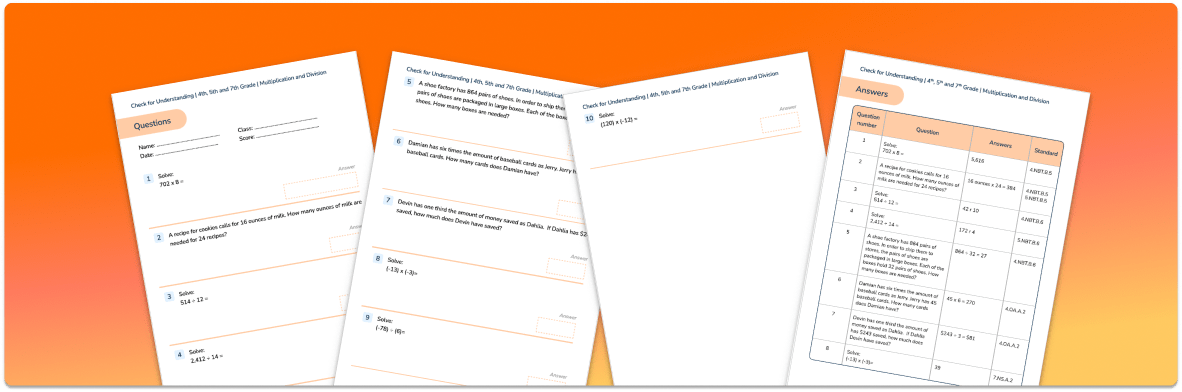Math Resources Quizzes

# Multiplication and Division Check for UnderstandingUse this quiz to check your grade 4, 5 and 7 students’ understanding of types of data.

• 10 questions with answers covering a range of 4, 5 and 7 grade types of data topics.
• Identify areas of strength and where support is needed with topics such as multiplying 3-digit numbers by single digit numbers, dividing up to 4-digit numbers by up to 2-digit numbers and worded problems.

You can unsubscribe at any time (each email we send will contain an easy way to unsubscribe). To find out more about how we use your data, see our privacy policy.

## Personalized one-on-one math instruction made easy

At Third Space Learning, we’re on a mission to help teachers like you shape your students into confident, able mathematicians.

Request a demo